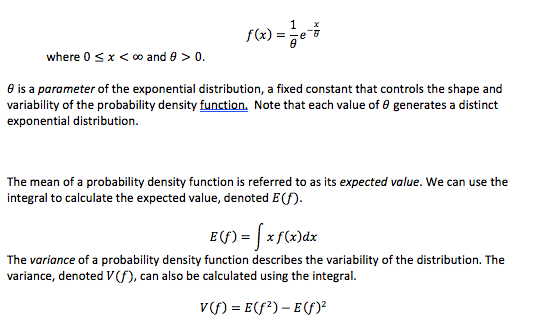Question
2 views

# How are the mean and variance of an exponential random variable related? *requires integration by parts? More information is given in the picture but this is the question i am trying to answer. I really would appreciate clean handwriting in the answer as a lot of answers and variables are too difficult for me to followhelp_outlineImage Transcriptionclosewhere 0 x < oo and 0> 0. e is a parameter of the exponential distribution, a fixed constant that controls the shape and variability of the probability density function. Note that each value of e generates a distinct exponential distribution The mean of a probability density function is referred to as its expected value. We can use the integral to calculate the expected value, denoted E(f) E(f)xf(x)dx The variance of a probability density function describes the variability of the distribution. The variance, denoted V(f), can also be calculated using the integral v) Ef2)-E) fullscreen
check_circle

Step 1

Introduction:

For any continuous random variable, X with probability density function f (x), the expectation, E...

### Want to see the full answer?

See Solution

#### Want to see this answer and more?

Solutions are written by subject experts who are available 24/7. Questions are typically answered within 1 hour.*

See Solution
*Response times may vary by subject and question.
Tagged in

### Other